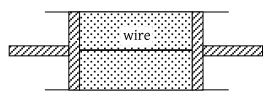A mono atomic gas is supplied the heat Q very slowly keeping the pressure constant. The work done by the gas will be

(1) $\frac{2}{3}Q$

(2) $\frac{3}{5}Q$

(3) $\frac{2}{5}Q$

(4) $\frac{1}{5}Q$

Concept Questions :-

Molar Specific heat
High Yielding Test Series + Question Bank - NEET 2020

Difficulty Level:

A gas mixture consists of 2 moles of oxygen and 4 moles argon at temperature T. Neglecting all vibrational modes, the total internal energy of the system is

(1) 4 RT

(2) 15 RT

(3) 9 RT

(4) 11 RT

Concept Questions :-

Basic terms
High Yielding Test Series + Question Bank - NEET 2020

Difficulty Level:

An ideal gas expands isothermally from a volume V1 to V2 and then compressed to original volume V1 adiabatically. Initial pressure is P1 and final pressure is P3. The total work done is W. Then -

(1) ${P}_{3}>{P}_{1},\text{\hspace{0.17em}\hspace{0.17em}}W>0$

(2) ${P}_{3}<{P}_{1},\text{\hspace{0.17em}\hspace{0.17em}}W<0$

(3) ${P}_{3}>{P}_{1},\text{\hspace{0.17em}\hspace{0.17em}}W<0$

(4) ${P}_{3}={P}_{1},\text{\hspace{0.17em}\hspace{0.17em}}W=0$

Concept Questions :-

Work done by gas
High Yielding Test Series + Question Bank - NEET 2020

Difficulty Level:

Work done by a system under isothermal change from a volume V1 to V2 for a gas which obeys Vander Waal's equation $\left(V-\beta n\right)\text{\hspace{0.17em}}\left(P+\frac{\alpha {n}^{2}}{V}\right)=nRT$

(1) $nRT{\mathrm{log}}_{e}\left(\frac{{V}_{2}-n\beta }{{V}_{1}-n\beta }\right)+\alpha \text{\hspace{0.17em}}{n}^{2}\text{\hspace{0.17em}}\left(\frac{{V}_{1}-{V}_{2}}{{V}_{1}{V}_{2}}\right)$

(2) $nRT{\mathrm{log}}_{10}\left(\frac{{V}_{2}-\alpha \beta }{{V}_{1}-\alpha \beta }\right)+\alpha \text{\hspace{0.17em}}{n}^{2}\text{\hspace{0.17em}}\left(\frac{{V}_{1}-{V}_{2}}{{V}_{1}{V}_{2}}\right)$

(3) $nRT{\mathrm{log}}_{e}\left(\frac{{V}_{2}-n\alpha }{{V}_{1}-n\alpha }\right)+\beta \text{\hspace{0.17em}}{n}^{2}\text{\hspace{0.17em}}\left(\frac{{V}_{1}-{V}_{2}}{{V}_{1}{V}_{2}}\right)$

(4) $nRT{\mathrm{log}}_{e}\left(\frac{{V}_{1}-n\beta }{{V}_{2}-n\beta }\right)+\alpha \text{\hspace{0.17em}}{n}^{2}\text{\hspace{0.17em}}\left(\frac{{V}_{1}{V}_{2}}{{V}_{1}-{V}_{2}}\right)$

Concept Questions :-

Work done by gas
High Yielding Test Series + Question Bank - NEET 2020

Difficulty Level:

A cylindrical tube of uniform cross-sectional area A is fitted with two air tight frictionless pistons. The pistons are connected to each other by a metallic wire. Initially the pressure of the gas is P0 and temperature is T0, atmospheric pressure is also P0. Now the temperature of the gas is increased to 2T0, the tension in the wire will be(1) 2 P0A

(2) P0A

(3) $\frac{{P}_{0}A}{2}$

(4) 4 P0A

Concept Questions :-

Types of processes
High Yielding Test Series + Question Bank - NEET 2020

Difficulty Level:

The molar heat capacity in a process of a diatomic gas if it does a work of $\frac{Q}{4}$ when a heat of Q is supplied to it is -

(1) $\frac{2}{5}R$

(2) $\frac{5}{2}R$

(3) $\frac{10}{3}R$

(4) $\frac{6}{7}R$

Concept Questions :-

Molar Specific heat
High Yielding Test Series + Question Bank - NEET 2020

Difficulty Level:

An insulator container contains 4 moles of an ideal diatomic gas at temperature T. Heat Q is supplied to this gas, due to which 2 moles of the gas are dissociated into atoms but temperature of the gas remains constant. Then

(1) Q = 2RT

(2) Q = RT

(3) Q = 3RT

(4) Q = 4RT

Concept Questions :-

First law of thermodynamics
High Yielding Test Series + Question Bank - NEET 2020

Difficulty Level:

The volume of air increases by 5% in its adiabatic expansion. The percentage decrease in its pressure will be -

(1) 5%

(2) 6%

(3) 7%

(4) 8%

Concept Questions :-

Types of processes
High Yielding Test Series + Question Bank - NEET 2020

Difficulty Level:

The temperature of a hypothetical gas increases to $\sqrt{2}$ times when compressed adiabatically to half the volume. Its equation can be written as

(1) PV3/2 = constant

(2) PV5/2 = constant

(3) PV7/3 = constant

(4) PV4/3 = constant

Concept Questions :-

Types of processes
High Yielding Test Series + Question Bank - NEET 2020

Difficulty Level:

Two Carnot engines A and B are operated in succession. The first one, A receives heat from a source at T1 = 800 K and rejects to sink at T2 K. The second engine B receives heat rejected by the first engine and rejects to another sink at T3 = 300 K. If the work outputs of two engines are equal, then the value of T2 is -

(1) 100K

(2) 300K

(3) 550K

(4) 700K

Concept Questions :-

Heat engine and refrigerator